### The very beginning Basics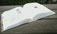InfoTopic: When you start the app your iThing becomes a calculator, and you can refer to the screen as you read this description (tap the yellow key then tap WEB to toggle from the live app to this Guide, tap MAIN to toggle back to the live app): The opening screen looks like Fig. 1: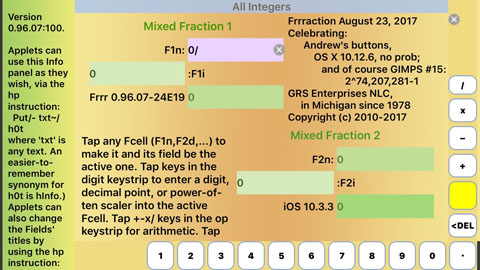Figure 1Frrraction's opening screen. This is Frrraction's MAIN view. It contains seven regions: The two numeric fields, presently fraction F1 and fraction F2 The display field called hShow in the upper right quadrant The program notes field called hNote in the lower left quadrant The keystrip along the bottom and righthand edge of the screen The narrow information display field at the screen's left edge, called hInfo The blue MAIN title label along the top of the screen. When Frrraction first opens, the six rectangular cells within the fraction fields—one white, five greenish—are the integer, numerator and denominator parts of two mixed fractions. Both fractions have been initialized to contain numerically indeterminate fractions: 0 + ( 0 over 0 ) The symbol '/' seen in F1n as '0/' in the screenshot is Frrraction's digit-cursor which in realtime alternates between | and / looking like a single waving stroke, to show where the next input digit will go. Tapping any of the green fCells moves the digit-cursor and the white background to the one you tapped, making it become "the active cell". The role of the white keys along the bottom of the screen is number entry. The white keys along the right edge of the screen trigger arithmetic operations. The yellow key exposes the rest of Frrraction's power and is described a bit later in a section of its own within this guide.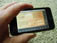TryIt: (starting with 0+0/0 in F1) with F1n active: Tap the key whose label is the digit 5. See where the digit went? See where the cursor is now? The little circled × in F1n is the cell's "clear button" or "Clr button". Each fCell has its own Clr button, visible only when the cell is active. Tapping a cell's Clr clears the cell to the integer zero. Tap F1n's Clr button. Now F1 contains 0+0/0 again. Finish this TryIt by loading 5 into F1n again.TryIt: (starting with 0+5/0 in F1) and F1n active: Tap the 8-key. Since 5 was already present, tapping 8 left the numeric value 58 in F1n. Next tap the green F1d key, then put the integer 82 into F1d by tapping the 8-key then the 2-key. Now F1 contains the pure fraction 0+58/82. Finish this TryIt by putting the integer 3 into F1i (tap the green F1i cell, then tap the 3-key), so F1 contains the mixed fraction 3+87/123

### A numbers-math app for iPhone and iPad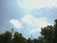Things to find out: Math is not a passive subject. Frrraction helps you work with numbers in the relaxed atmosphere of your own handheld number-support system. What's the biggest integer an iPhone can handle? [This became a trick question when the iPhone 5S came out.] What's the biggest pure fraction that Frrraction can handle? What if you add 1 to make the biggest fraction bigger? What if you divide a number by 0? What if you divide 0 by 0? How do you find simple little fractions like 22/7 to replace big awkward fractions like 314159/100000? Given decimal fractions like 3.14159 how do you find cute little pure fractions like 22/7 to stand in their place? Are mixed fractions more general than pure fractions? Integers are not fractions, but can fractions equal integers? How do you know which integers are prime? If an integer is not prime, what are its prime factors? When is 5 + 9 = 3? and 5 * 9 = 1? (Hint: In modular arithmetic when the modulus is 11.) What are "continued fractions"? What are they good for? Some All this and more you can find out for yourself with Frrraction. And when you do? Mathematics is a big world. As they say, the sky's the limit!Fraction representations: Foremost among them are the pure ratio fraction (formatted as two integers:    num and den separated by a division symbol), e.g. 456/123 the mixed ratio fraction (formatted as three integers:    integer, num and den separated by a plus and a division symbol), e.g. 3 + 58/82 and the dot fractionalso known rather misleadingly as the decimal fraction(formatted as two integers:    integer part and fractional part separated by a decimal point) e.g. 3.707317 Two other fraction formats favored by Frrraction are: continued fraction, e.g.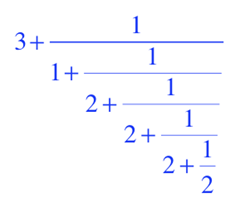and egyptian fraction, e.g.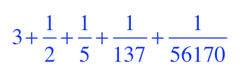A point of interest: The five examples just given are different forms of the same fraction. All but the dot-form are exact, while the dot form is an approximation. A more precise dot form is 3.7073170731707317073170731. But even that one is still inexact.